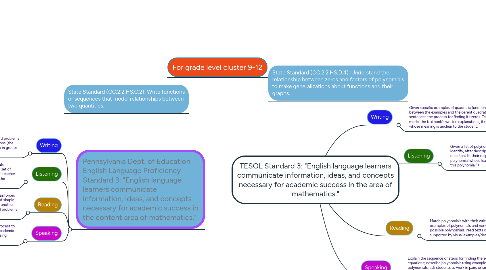# TESOL Standard 3: "English language learners communicate information, ideas, and concepts necessa...Alex Muchow

Get Started. It's FreeTESOL Standard 3: "English language learners communicate information, ideas, and concepts necessary for academic success in the area of mathematics."## 1. Pennsylvania Dept. of Education English Language Proficiency Standard 3: "English language learners communicate information, ideas, and concepts necessary for academic success in the content area of mathematics."

### 1.1. Writing

1.1.1. Students translate equations of two variables into word problems or real-life situations that could describe those equations (the opposite process in the "Reading" skill). Could be done in groups of 2 if necessary

### 1.2. Listening

1.2.1. The teacher orally poses a few problems on how to manipulate functions of two variables, requesting that students follow a set of steps to arrive at a new function. When steps are completed, teacher asks students to tell him how the new function differs from the original.

1.2.1.1. Example of such a problem: "Add one to the right side of the equals sign in problem #5. Create a table of x and y values that shows how the new function differs from the original one. Choose three appropriate x values. Ask me for help if necessary."

1.3.1. After slowly and carefully modeling an example, in which the teacher reinforces key vocabulary from the lesson, he instructs students to read a series of simple word problems wherein one quantity, such as time, varies directly with another quantity, such as distance. Then he asks students to translate the word problems into equations. Could be done in groups of 2 if necessary.

1.3.1.1. Example of such a problem: "The Robinson family drives 70 miles in 2 hours. If they continue at this rate, how many miles will the family drive in x hours?"

### 1.4. Speaking

1.4.1. Students are asked to orally explain their thought process to solving a problem. Teacher encourages the use of academic vocabulary and asks other students to fill in the missing steps/gaps in the explanation.

## 5. Speaking

### 5.1. Explain the sequence of steps for finding the zeroes of polynomial equations; describe polynomials using examples from a list of polynomials; ask students to work in pairs or small groups creating a graph of a polynomial equation and explaining to the rest of the class its properties.

5.1.1. I have already had success in implementing an activity like Example 3, where students work in small groups and describe the properties of polynomials, with two EL students in my Algebra class. After that mini-project and reinforced support in group and individual activities, those two students scored significantly higher on the summative assessment I gave them. Each of them worked with a partner who was not an EL learner.